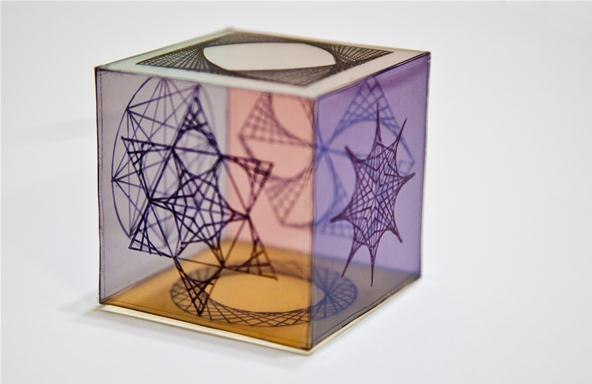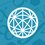# Colouring a CUBE !!!I got this problem from a friend and couldn't solve it ...

Imagine a cube which needs to be painted with different colors on all it's faces.

In how many different ways can I do this if I have 6 different colors ?Note by Santanu Banerjee
6 years, 9 months ago

This discussion board is a place to discuss our Daily Challenges and the math and science related to those challenges. Explanations are more than just a solution — they should explain the steps and thinking strategies that you used to obtain the solution. Comments should further the discussion of math and science.

When posting on Brilliant:

• Use the emojis to react to an explanation, whether you're congratulating a job well done , or just really confused .
• Ask specific questions about the challenge or the steps in somebody's explanation. Well-posed questions can add a lot to the discussion, but posting "I don't understand!" doesn't help anyone.
• Try to contribute something new to the discussion, whether it is an extension, generalization or other idea related to the challenge.

MarkdownAppears as
*italics* or _italics_ italics
**bold** or __bold__ bold
- bulleted- list
• bulleted
• list
1. numbered2. list
1. numbered
2. list
Note: you must add a full line of space before and after lists for them to show up correctly
paragraph 1paragraph 2

paragraph 1

paragraph 2

[example link](https://brilliant.org)example link
> This is a quote
This is a quote
    # I indented these lines
# 4 spaces, and now they show
# up as a code block.

print "hello world"
# I indented these lines
# 4 spaces, and now they show
# up as a code block.

print "hello world"
MathAppears as
Remember to wrap math in $$ ... $$ or $ ... $ to ensure proper formatting.
2 \times 3 $2 \times 3$
2^{34} $2^{34}$
a_{i-1} $a_{i-1}$
\frac{2}{3} $\frac{2}{3}$
\sqrt{2} $\sqrt{2}$
\sum_{i=1}^3 $\sum_{i=1}^3$
\sin \theta $\sin \theta$
\boxed{123} $\boxed{123}$

Sort by:

Presumably you would regard two cube colourings the same if one can be obtained from the other by rotation.

When counting colourings, start by rotating all the colourings so that the red face is on the bottom. There are clearly $5$ choices for the colour of the top face, opposite the red one.

There are at first sight $4!=24$ colourings of the four side faces. However, since we can still spin the cube about its vertical axis (and maintain the red face at the bottom), these $24$ colourings come in $6$ groups of four, where the colourings in each group of four are essentially identical.

Thus we have $5$ choices for the colour of the face opposite red, and then $6$ ways to colour the other four faces. There are $30$ different colourings.

How does this relate to $6!=720$? There are exactly $24$ rotations of the cube (the "do-nothing" rotation, $6$ rotations through $90^\circ$ about axes through the midpoints of opposite faces, $3$ rotations through $180^\circ$ about axes through the midpoints of opposite faces, $6$ rotations through $180^\circ$ about axes through midpoints of opposite edges and $8$ rotations through $120^\circ$ about axes through pairs of opposite vertices.

If you take any colouring, there are a total of $24$ positions into which that colouring can be rotated, and so $24$ of the $720$ are essentially the same colouring. Thus there are $720 \div 24 \,=\,30$ essentially different colourings.

- 6 years, 9 months ago

Seems legit.

- 6 years, 9 months ago

I am just wondering, how come there are $24$ rotations, according to my calculation it should be $12$. Let's consider we are rotating the cube about the top edge, its also rotating about bottom edge at the same time, whichever direction you rotate. Meaning, we are getting $4$ rotated distinct configurations, for rotating $2$ sides at the same time. And hence, the total no. of position should be $3*4=12$. And if I am right, the corresponding answer should be $720\div12=\fbox{60}$

- 6 years, 9 months ago

I have described all $24$ rotations.

What do you mean by "top edge"?

I think you mean rotations about the (axis through the midpoints of the) top and bottom faces. For each of the three possible axes, there are indeed four rotations (through $0^\circ,90^\circ,180^\circ,270^\circ$. But the rotation through $0^\circ$ is the "do-nothing" rotation, which is the same rotation for each of the three choices of axis. Thus you are triple-counting this rotation. Your count has found the identity ("do nothing") rotation, the $6$ rotations through $\pm90^\circ$ about the axes through midpoints of opposite faces, and the $3$ rotations through $180^\circ$ about the same axes. Thus we have found just $10$ (not $12$, because of the triple-counting of the identity). However, you have missed the other two types of rotation, about axes through midpoints of opposite pairs of edges, and about axes through opposite faces.

- 6 years, 9 months ago

- 6 years, 9 months ago

It's $6!/24$. There are $6!$ ways in which a cube held in particular position be colored. But there are exactly $24$ positions which resemble the same color configuration if cube rotated accordingly. A cube can be placed on $6$ different sides and then can be rotated $4$ times horizontally by keeping the top face same and $24 (= 6 * 4)$ different positions be achieved of the same color configuration. Hence the number of ways $6!/24$.

- 6 years, 9 months ago

this is an easier way of counting the 24 cube rotations.

- 6 years, 8 months ago

I got it at last...

First I will consider a face to be fixed with a specific colour and find out the relative arrangements of the rest.

When I have fixed a colour , the opposite face can be painted in the rest 5 colours , thus there are 5 choices...

Now the rest four face I can calculate using the circular arrangements concept

Similar to Permutations on a Circular Table...

That is 4 colours can be painted on the remaining 4 faces in ( 4 - 1 ) !

Thus the answer should be 5 * ( 4 - 1 ) ! == 30

- 6 years, 9 months ago

Thanks a lot....this is a much better way of doing it than thinking about the 24 possible ways in which a cube can be rotated to give the same set

- 6 years, 2 months ago

But I thought of an alternative way...which probably is actually the same thing... 1. Fix one side 2. Using 5C4 select 4 out of the remaining 5 colours 3. Arrange them in a circular pemutation in 3! ways 4. The remaining color automatically occupies the side opposite to our original color... ANS: 5C4*3!=30 Its just that though I accepted your way...I needed to be sure of my thinking!!

- 6 years, 1 month ago

If you want a tough problem, how many colourings are possible (up to rotation) if there are still $6$ colours, but they can be repeated (so you could have a colouring with four red faces, one white and one green, for example)?

- 6 years, 9 months ago

You can paint the two Opposite faces in 6*5=30 ways.The order of the rest of the coloring does not matter.Sweet and Simple.

- 6 years, 8 months ago

Guys...can u please explain the 24 rotations of the cube that give the same set...I didnt quite understand it...Thanks in Advance!!

- 6 years, 1 month ago

Here's the fancy way to do problems like these: Burnside's Lemma AoPS also has an introduction to this technique.

- 6 years, 9 months ago

Excellent idea. And the answer to my supplementary question is... ?

- 6 years, 9 months ago

What happens if it's a 12 Faced Polygon

and we have 12 predefined colours. No colour repeated ...

- 6 years, 9 months ago

$2226$: yes.

The dodecahedron has rotation group of order $60$ ((a) six axes through midpoints of opposite faces, four nontrivial rotations each, (b) fifteen axes through midpoints of opposite edges, one nontrivial rotation each, (c) 10 axes through pairs of opposite vertices, two nontrivial rotations each, (d) the identity. $6\times4 + 15\times1 + 10\times2 + 1 = 60$).

If we have twelve colours, and none are repeated, there are $\tfrac{12!}{60} = 7983360$ different colourings.

And if colours can be repeated ...?

- 6 years, 8 months ago

- 6 years, 9 months ago

Possibly. However, in the original problem, the orbits all have the same size ($24$), so counting them by Burnside's Lemma is not necessary.

- 6 years, 9 months ago

As the Cube is symmetrical,paint the top side with any one of the six colors.Then the adjacent 4 sides can be permuted in 3! ways (circular permutation) and respective colors in {5 \choose 4} ways which makes it 30.And paint the remaining side with the remaining color.Hence my answer is 30.

- 6 years, 9 months ago

If all the faces of cubes are identical than there is only one way but if all thhe faces are distinct than there are 6! Ways

- 6 years, 9 months ago

if you want that each side has a different color each side calling A, B, C, D, E, F on side A will have 6 color options of which only one is needed for the B side will have 5 colors remaining for C will have 4 for D will have 3 for E will have 2 and for F only stay one color: that according to the principle of multiplication would be: 6! : 6x5x4x3x2x1 720 possible combinations

- 6 years, 8 months ago

Don't forget that if you rotate a cube with a certain colouring, it stays the same cube.

- 6 years, 8 months ago

if one of the side is painted with one color than we have 5 color left and than if paint 2 sides we have 4 colors and so on ...... so,it will be 6! i.e. 65432*1=720

- 6 years, 8 months ago

No it wont be..Each of unique coloring will be repeated 24 Times !!!

- 6 years, 8 months ago

The word "different" is the key here. First consider that every face has a fixed orientation in space. Then definitely you can color them "differently" in $6!$ ways. Now if the faces do not have fixed orientation (that is exactly what is meant here) then we do not have that many different colorings. Because if we obtain same configuration of face-colorings in space by appropriately rotating different colorings, then those colorings are considered to be the "same". Hence the central question is how many different "rotational symmetries" a cube possesses. The answer is non-trivial and is 24. Hence number of different colorings for a cube is $6!/24=30$.

- 6 years, 2 months ago

...6!/24 IS IT RIGHT?

- 5 years, 4 months ago

But I think it should be less than 6! since it can be rotated about a central axis which gives 4 same combinations for the lateral faces. We can choose the first 4 colours by 6C4 ways. Now these 4 colours can be arranged in (4-1)! ways since there are 4 same combinations for the lateral faces. The last two colours can be selected only in one way as it can be seen in an upside-down way too. Hence, the number of ways may be 6C4 x 3!, but I still have a doubt whether the cube can be seen in some way as well.

- 6 years, 9 months ago